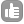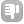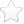0This is my first time in doing DOSY experiment. i got the data but dont know how to process it. asked Jun 12 '12 at 01:171Dear Kiu, Mestrenova will do nice 2D plots of Dosy for you, what helps a lot to identify different substances. If you are interested in checking the quality of your fit however, you better get the data points from MestreNova and plot it yourself (intensities Vs gradients plot, I mean). I have experienced some strange behaviour of topspin distributing data points in incomprehensible manner. Thus if you use topspin you better plot the data in a math program as well. There is no other way to know if you have a reasonable fit or a curve trying to fit crazy points. (obs: VnmrJ does show you the fit graph). When doing the fit, check if the points are deviating in a random manner from the fitted curve, discard your experiment otherwise. Dosy expts are quite sensitive to conditions and may easily give wrong results. Maybe here is a suggestion for Mestrenova to plot the fit (Echo Intensities Vs Gradient Intensities) as well. It would be a nice feature to help us find out why different experiments give completely different results :-) And believe me, it happens quite often. Below I show you how I fitted a Dosy of DMSO using the program Octave (additional function leasqr required): % comments start with a % % xydata= gradients in %, echo intensities xydata=[ 10                  6.21843e+06 13.3333          5.53812e+06 16.6667         4.49104e+06 20                 4.0029e+06 23.3333         3.22418e+06 26.6667          2.49896e+06 30                  1.8643e+06 33.3333          1.33617e+06 36.6667          923999 40                  613558 43.3333          393992 46.6667          244480 50                  145317 53.3333          83411.1 56.6667          46831.3 60                  38571.2 ]; gcal=42.547; % Gauss/m p1=11.2e-6; p2=22.4e-6; p19=1000e-6; p30=1000e-6; d16=0.0003; d20=0.4; nuclgamma= 2.675222005e4; % rad / (s Gauss) k=1.3806503e-23; % m2 kg s-2 K-1 T=298.15; eta=1.991e-3 ; % viscosity of dmso legend='dmso'; delta = 2p30 ; DELTA = d20; exponent_cnst= (nuclgammadelta)^2 * (DELTA -delta/3 - d16/2); xydata=[xydata(:,1)gcal xydata(:,2)/max(xydata(:,2))]; p2guess=(log(xydata(1,2)/xydata(end,2))/(xydata(end,1)^2 - xydata(1,1)^2)); p1guess=xydata(1,2)/exp(-p2guessxydata(1,1)^2); pguess=[p1guess p2guess]; fitfunc=@(x,p) p(1)exp(-p(2)x.^2); [f,coef,cvg,iter,corp,covp,covr,stdresid,Z,r2]=... leasqr(xydata(:,1),xydata(:,2),pguess, fitfunc); D=coef(2)/exponent_cnst; Rh=kT/(6pietaD); printf("\n\n=== Your Diff coef is %g, Rh=%g ==== \n \n", D , Rh) %----------------- ploting ------------------------- clf plot(xydata(:,1),xydata(:,2), 'o', 'markersize', 16) hold on lineover=0.05; linemin=min(xydata(:,1))-lineoverrange(xydata(:,1)); linemax=max(xydata(:,1))+lineoverrange(xydata(:,1)); x=(linemin:range(xydata(:,1))/1000:linemax)'; plot(x, fitfunc(x, coef),'-', 'linewidth', 3) border=0.1; Gmin=min(xydata(:,1))-borderrange(xydata(:,1)); Gmax=max(xydata(:,1))+borderrange(xydata(:,1)); Emin=min(xydata(:,2))-borderrange(xydata(:,2)); Emax=max(xydata(:,2))+borderrange(xydata(:,2)); axis([Gmin Gmax Emin Emax]) xlabel('Gradient / Gauss m^{-1}') ylabel('Echo Intensity') answered Jun 16 '12 at 05:44fid 1391Hey, sorry. Have to post the code again. This wiki is using markdown. It changed a little bit what i had typed ... `````` % xydata= gradients in %, echo intensities xydata=[ 10 6.21843e+06 13.3333 5.53812e+06 16.6667 4.49104e+06 20 4.0029e+06 23.3333 3.22418e+06 26.6667 2.49896e+06 30 1.8643e+06 33.3333 1.33617e+06 36.6667 923999 40 613558 43.3333 393992 46.6667 244480 50 145317 53.3333 83411.1 56.6667 46831.3 60 38571.2 ]; gcal=42.547; % Gauss/m p1=11.2e-6; p2=22.4e-6; p19=1000e-6; p30=1000e-6; d16=0.0003; d20=0.4; nuclgamma= 2.675222005e4; % rad / (s Gauss) k=1.3806503e-23; % m2 kg s-2 K-1 T=298.15; eta=1.991e-3 ; % viscosity of dmso delta = 2*p30 ; DELTA = d20; exponent_cnst= (nuclgamma*delta)^2 * (DELTA -delta/3 - d16/2); xydata=[xydata(:,1)*gcal xydata(:,2)/max(xydata(:,2))]; p2guess=(log(xydata(1,2)/xydata(end,2))/(xydata(end,1)^2 - xydata(1,1)^2)); p1guess=xydata(1,2)/exp(-p2guess*xydata(1,1)^2); pguess=[p1guess p2guess]; fitfunc=@(x,p) p(1)*exp(-p(2)*x.^2); [f,coef,cvg,iter,corp,covp,covr,stdresid,Z,r2]=... leasqr(xydata(:,1),xydata(:,2),pguess, fitfunc); D=coef(2)/exponent_cnst; Rh=k*T/(6*pi*eta*D); printf("\n\n=== Your Diff coef is %g, Rh=%g ==== \n \n", D , Rh) %----------------- ploting ------------------------- clf plot(xydata(:,1),xydata(:,2), 'o', 'markersize', 16) hold on lineover=0.05; linemin=min(xydata(:,1))-lineover*range(xydata(:,1)); linemax=max(xydata(:,1))+lineover*range(xydata(:,1)); x=(linemin:range(xydata(:,1))/1000:linemax)'; plot(x, fitfunc(x, coef),'-', 'linewidth', 3) border=0.1; Gmin=min(xydata(:,1))-border*range(xydata(:,1)); Gmax=max(xydata(:,1))+border*range(xydata(:,1)); Emin=min(xydata(:,2))-border*range(xydata(:,2)); Emax=max(xydata(:,2))+border*range(xydata(:,2)); axis([Gmin Gmax Emin Emax]) xlabel('Gradient / Gauss m^{-1}') ylabel('Echo Intensity') `````` answered Jun 16 '12 at 06:11fid 139 Hey fid, you could have just edited your post. Can you put together one answer instead of two and delete the other one? Thanksl - Evgeny Fadeev (Jun 16 '12 at 09:36)0Dear Kiu Chor Sham, Could you please take a look at this tutorial? If you have doubts, please contact us Regards answered Jun 12 '12 at 07:22